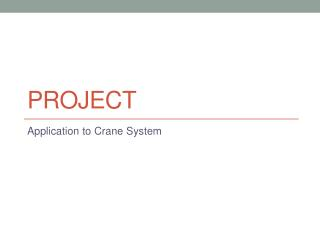DownloadDownload PresentationProJect

# ProJect

Télécharger la présentation## ProJect

- - - - - - - - - - - - - - - - - - - - - - - - - - - E N D - - - - - - - - - - - - - - - - - - - - - - - - - - -
##### Presentation Transcript

1. ProJect Application to Crane System

2. 1. Objective • Understand of application of inverted pendulum • To minimize the time and reduce the vibrations apply the PID controller to crane system as moves to a goal.

3. 2. Introduction • Crane system has 1 input and 2 outputs -> MIMO (Multi Input Multi Output) System • The inverted pendulum’s angle signal(Encoder) becomes signal of sensing the vibrations. • Cart’s location signal(Encoder) is used for sensing the location of packages. • There are no additional conditions to use inverted pendulum system. • It always needs to be downwards direction and stopped.

4. 3. Problem • 1. Modeling the following Crane system • Control the system using 2. PID, 3. Pole Placement

5. 4. Mission • Modeling the crane system • Control the system to locate following procedure. • Start at 0 position, after 5 seconds +10, after 15senconds +20, after 25 seconds -10 • Simulation the PID controller using the crane system equation • Simulation the Pole Place controller using the crane system equation • Experiment the PID controller • Experiment the Pole Place controller • Compare and analysis the results of simulation and experiment • Control the crane system has to be accurate and good performance • REPORT : 10 Points • Results of Experiments : 10 Points